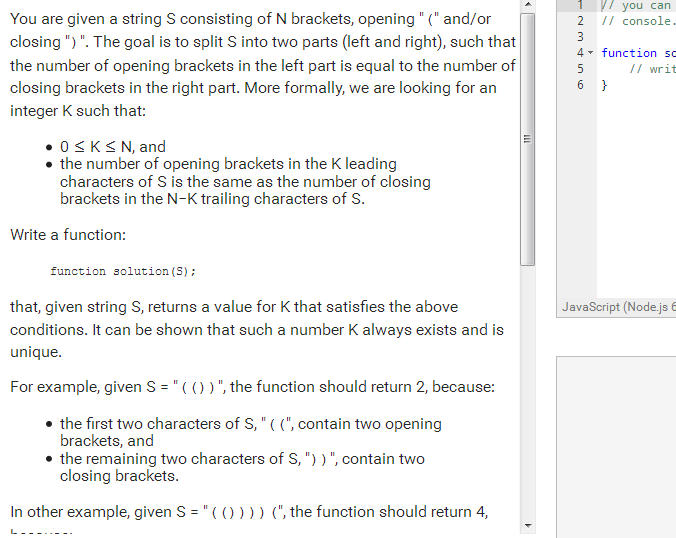# Codility brackets opening closing.

codility brackets opening closing.

codility you are given a string s consisting of n brackets opening problem solution.codility brackets opening closing

```function isBalanced(\$str){ \$count = 0; \$ocount = 0; \$ccount = 0; \$length = strlen(\$str); for(\$i = 0; \$i < \$length; \$i++){ if(\$str[\$i] == '(') \$ocount += 1; else if(\$str[\$i] == ')') \$ccount += 1; if(\$ccount == \$ocount){ \$count = \$ocount; }else{ \$count = \$ccount; } } return \$count; } echo isBalanced("))");```